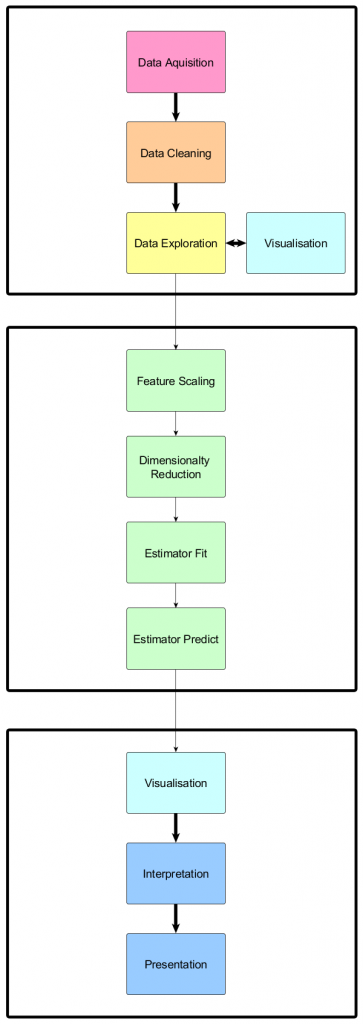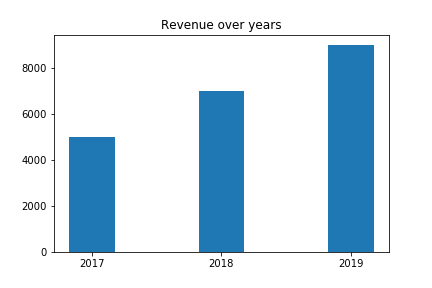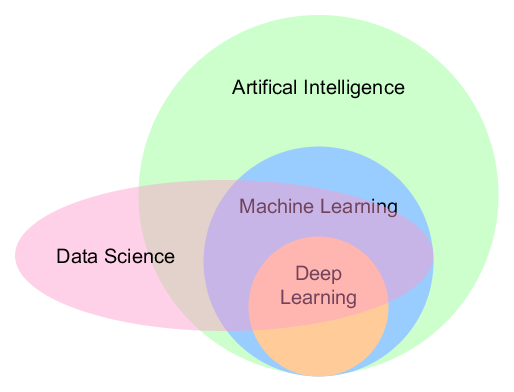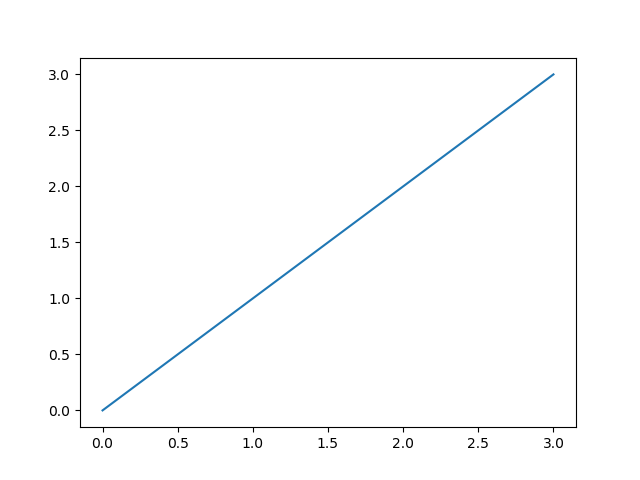## k-fold crossvalidation with sklearn

```from sklearn.model_selection import KFold

kf = KFold(n_splits=2)

kf.split(df_train)

step = 0 # set counter to 0
for train_index, val_index in kf.split(df_train): # for each fold
step = step + 1 # update counter

print('Step ', step)

features_fold_train = df_train.iloc[train_index, [4, 5]] # features matrix of training data (of this step)
features_fold_val = df_train.iloc[val_index, [4, 5]] # features matrix of validation data (of this step)

target_fold_train = df_train.iloc[train_index, 6] # target vector of training data (of this step)
target_fold_val = df_train.iloc[val_index, 6] # target vector of validation data (of this step)

print("VALIDATE:", val_index)
print('Dimensions features matrix for validation: ', features_fold_val.shape)
print("TRAIN:", train_index)
print('Dimensions features matrix for training: ',features_fold_train.shape, '\n')```

## Pandas Cheat Sheet

```import pandas as pd

## Data Cleaning

Dropping columns

`del df["column_name"]`

## Data Science Pipeline

Learning Data Science can be grueling and overwhelming sometimes. When I feel too overwhelmed it’s time to draw a picture. This my current overview of what a data scientist has to do:## SQLite3: Python and SQL

Everything we did in last articles was a dry run because we just used SQLFiddle. So let’s start with a real database like SQLite.

SQLite is a file based DBRMS and can be used for e.g. web sites. The official docs say:

“SQLite works great as the database engine for most low to medium traffic websites (which is to say, most websites). [..] Generally speaking, any site that gets fewer than 100K hits/day should work fine with SQLite.”

Because Knight Industries is not Google, Amazon nor Facebook we can definitely use SQLite.

## Creating and connecting to a database

In Python it is pretty easy to connect to a SQLite database:

```from sqlite3 import connect
db_connection = connect('knight_industries.db')```

If the file knight_industries.db does not exist, it will be created automagically. A nice little feature of the sqlite3 library.

But be careful: If You already have a database file and you mess up the path in the connect statement you will wonder why you cannot access your data, because a new file is created silently.

`cursor = db_connection.cursor()`
`cursor.execute('''CREATE TABLE operatives (id INTEGER, name TEXT, birthday DATE)''')`
```cursor.execute('''INSERT INTO operatives (id, name, birthday) \
VALUES (1, "Michael Arthur Long", "1949-01-09")''')

db_connection.commit()```
```cursor.execute('''SELECT * FROM operatives''')
print cursor.fetchone()
db_connection.close()```

## Introduction to matplotlib – Part 3

After laying the foundation in Introduction to matplotlib and Introduction to matplotlib – Part 2 I want to show you another important chart

## Bar Charts

A bar chart is useful to show total values over time e.g. the revenue of a company.

```years = (2017, 2018, 2019)
revenue = (5000, 7000, 9000)
plt.bar(years, revenue, width=0.35)
plt.xticks(years)
plt.title("Revenue over years")```Continue reading “Introduction to matplotlib – Part 3”

## Linear Regression with sklearn – cheat sheet

```# import and instantiate model
from sklearn.linear_model import LinearRegression
model = LinearRegression()

#prepare test data
features_train = df_train.loc[:, 'feature_name']
target_train = df_train.loc[:, 'target_name']

#fit (train) model and print coefficient and intercept
model.fit(features_train , target_train )
print(model.coef_)
print(model.intercept_)

# calculate model quality
from sklearn.metrics import mean_squared_error
from sklearn.metrics import r2_score

target_prediction = model.predict(features_train)
print(mean_squared_error(target_train , target_prediction))
print(r2_score(target_train , target_prediction))

# test predictions
features_test = df_train.loc[:, 'feature_name']
target_test = df_train.loc[:, 'target_name']
target_prediction_test = model.predict(features_test)
print(mean_squared_error(target_test, target_prediction_test ))
print(r2_score(target_test, target_prediction_test ))```

## 10 things I didn’t know about Data Science a year ago

In my article My personal road map for learning data science in 2018 I wrote about how I try to tackle the data science knowledge sphere. Due to the fact that 2018 is slowly coming to an end I think it is time for a little wrap up.

What are the things I learned about Data Science in 2018? Here we go:

## 1. The difference between Data Science, Machine Learning, Deep Learning and AIContinue reading “10 things I didn’t know about Data Science a year ago”

## Introduction to matplotlib

matplotlib is the workhorse of data science visualization. The module pyplot gives us MATLAB like plots.

The most basic plot is done with the “plot”-function. It looks like this:Continue reading “Introduction to matplotlib”

## Scatterplot with matplotlib

When you area already familiar with the basic plot from the introduction to matplotlib here is another type of plot used in data science.

A very basic visualization is the scatter plot:

```import numpy as np
import matplotlib.pyplot as plt

N = 100
x = np.random.rand(N)
y = np.random.rand(N)

plt.scatter(x, y)
plt.show()```